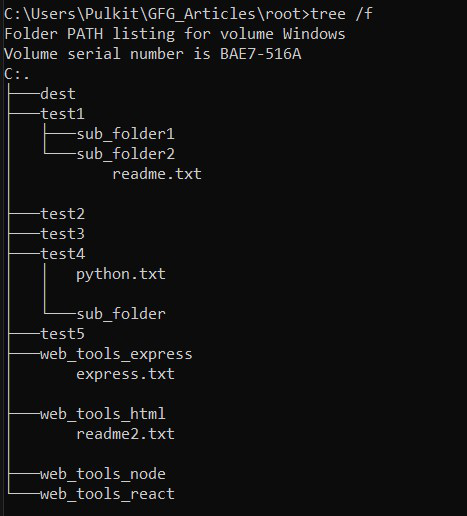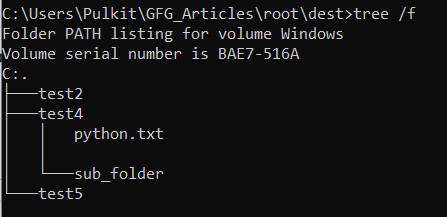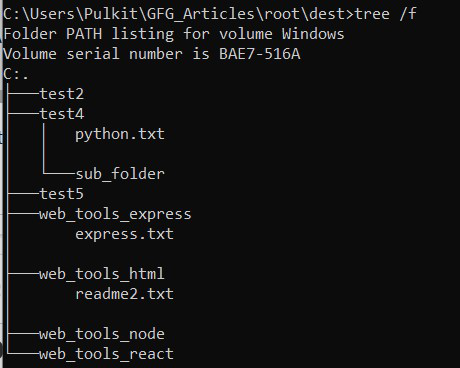# How to move list of folders with subfolders using Python ?

• Difficulty Level : Easy
• Last Updated : 24 Aug, 2022

Sometimes we need to move an entire directory or maybe there is a list of such directories say A along with its sub-content, files, and subfolders to another destination directory B. While this can be done manually by ‘cutting’ and ‘pasting’ but what if there are hundreds or thousands of directories you want to move let alone the human error! Let’s see how to do this easily in python with few lines of code using shutil module.

## The shutil module

The shutil provides a number of high-level functions that help in automating the process of copying, moving, or removal of files or directories irrespective of the platform used. It comes under Python’s standard utility modules, so there is no need for separate installation

It has a shutil.move() method which recursively moves a file or directory (source) along with its sub-content to another location (destination) and returns the destination. If the destination directory already exists then the source is moved inside that directory otherwise a new directory is created before moving. If the destination already exists but is not a directory then it may be overwritten or raise an error depending on os.rename() specifications.

Syntax: shutil.move(source, destination, copy_function = copy2)

Suppose the structure of the directory looks like this –where ‘dest’ is our destination folder.

## How to move list of folders with subfolders using Python ?

### Example 1: Based on a list of directories

Here we have made a list of directories we want to move. For the sake of simplicity, all the directories are in the same folder, you can also move from different base directories.

## Python3

 `# import shutil module` `import` `shutil`   `# import os module` `import` `os`   `# base path` `base_path ``=` `'C:/Users/Pulkit/GFG_Articles/root'`   `# list of directories we want to move.` `dir_list ``=` `[``'test2'``, ``'test4'``, ``'test5'``, ``'does_not_exist'``]`   `# path to destination directory` `dest ``=` `os.path.join(base_path, ``'dest'``)`   `print``(``"Before moving directories:"``)` `print``(os.listdir(base_path))`   `# traverse each directory in dir_list` `for` `dir_ ``in` `dir_list:`   `    ``# create path to the directory in the` `    ``# dir_list.` `    ``source ``=` `os.path.join(base_path, dir_)`   `    ``# check if it is an existing directory` `    ``if` `os.path.isdir(source):`   `        ``# move to destination path` `        ``shutil.move(source, dest)`   `print``(``"After moving directories:"``)` `print``(os.listdir(base_path))`

Output:

Before moving directories:

[‘dest’, ‘test1’, ‘test2’, ‘test3’, ‘test4’, ‘test5’, ‘web_tools_express’, ‘web_tools_html’, ‘web_tools_node’, ‘web_tools_react’]

After moving directories:

[‘dest’, ‘test1’, ‘test3’, ‘web_tools_express’, ‘web_tools_html’, ‘web_tools_node’, ‘web_tools_react’]

Let’s check the destination folder, which is as:As you can see we moved the entire directories and their contents to the destination directory (absolute path points to ‘dest’ directory).

### Example 2: Based on the pattern

Suppose we want to move directories that follow a specific name pattern to our destination. Let’s move all directories whose name starts with ‘web’. You can use any pattern according to your needs. This would be a more practical use case where we have hundreds of directories.

## Python3

 `# import shutil module` `import` `shutil`   `# import os module` `import` `os`   `# base path` `base_path ``=` `'C:/Users/Pulkit/GFG_Articles/root'`   `# get all directories in our base path.` `all_dir ``=` `os.listdir(base_path)`   `# path to destination directory` `dest ``=` `os.path.join(base_path, ``'dest'``)`   `print``(``"Before moving directories:"``)` `print``(os.listdir(base_path))`   `for` `dir_ ``in` `all_dir:`   `    ``# check if the dir_ follows the required` `    ``# pattern.` `    ``if` `dir_.startswith(``'web'``):`   `        ``# create path to this directory.` `        ``source ``=` `os.path.join(base_path, dir_)`   `        ``# move to destination path` `        ``shutil.move(source, dest)`   `print``(``"After moving directories:"``)` `print``(os.listdir(base_path))`

Output:

Before moving directories:

[‘dest’, ‘test1’, ‘test3’, ‘web_tools_express’, ‘web_tools_html’, ‘web_tools_node’, ‘web_tools_react’]

After moving directories:

[‘dest’, ‘test1’, ‘test3’]

Let’s check the destination folder, which is as:## Python3

 `# import shutil module` `import` `shutil` `# import os module` `import` `os` `# base path` `base_path ``=` `'C:/Users/Pulkit/GFG_Articles/root'` `# get all directories in our base path.` `all_dir ``=` `os.listdir(base_path)` `# path to destination directory` `dest ``=` `os.path.join(base_path, ``'dest'``)` `if` `not` `os.path.exists(dest):` `    ``os.mkdir(dest)`   `print``(``"Before moving directories:"``)` `print``([x ``for` `x ``in` `os.listdir(base_path) ``if` `os.path.isdir(os.path.join(base_path, x))])` `for` `dir_ ``in` `all_dir:` `    ``# check if the dir_ follows the required has been created before 7 days.` `    ``file_path ``=` `os.path.join(base_path, dir_)` `    ``if` `os.path.isdir(file_path):` `        ``c_timestamp ``=` `os.path.getctime(file_path)` `        ``file_create_time ``=` `datetime.fromtimestamp(os.stat(file_path).st_ctime)` `        ``if` `(datetime.today()``-``file_create_time).total_seconds() ``/` `24``*``3600` `> ``7``:      ` `            ``# create path to this directory.` `            ``source ``=` `os.path.join(base_path, dir_)` `            ``# move to destination path` `            ``shutil.move(source, dest)` `print``(``"After moving directories:"``)` `print``([x ``for` `x ``in` `os.listdir(base_path) ``if` `os.path.isdir(os.path.join(base_path, x))])`

Output:

```Before moving directories:
['btechmarksheet_files', 'chromedriver_win32 (1)', 'dest', 'How to make a fully transparent window with PyGame _-Write_files', 'gfg', 'tuhin • gfg photos and videos_files']
After moving directories:
['dest']```

Explanation:

Here we have calculated the difference between the directory creation time and todays date, and if the difference is greater than7 days then we can move that directory to the destination otherwise ignore that.

My Personal Notes arrow_drop_up
Recommended Articles
Page :